# Mathematics - All About Geometry (Part 3)

in StemSociallast month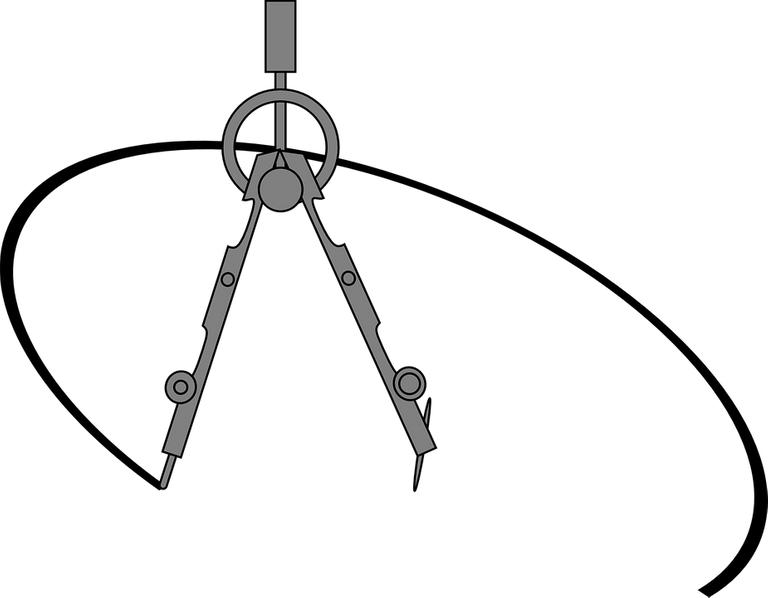[Image1]

## Introduction

Hey it's a me again @drifter1!

This is the third part of my high-school refresher series on Geometry.
I suggest checking out part1 and part2 before this.

So, without further ado, let's get straight into it!

## Cartesian Coordinate System

In order to easily specify where exactly a point or shape resides, we've defined various coordinate systems. Rectangular coordinates, such as the Cartesian Coordinate System, are the simplest format.

### Number Line

Let's first start with a single dimension, a line. Numbers can be written down on a so called Number Line, increasing right-wards and decreasing left-wards. Positive numbers are thus to the right of 0, whilst negative numbers are to the left of 0, as shown below.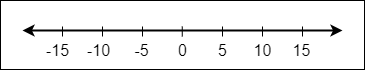The distance from zero is also called an absolute value. For example, 5 and -5 have both an absolute value of 5, or |5| = |-5| = 5, where wrapping in | | denotes the absolute value.

### Axes and Origin

On a plane, we use Cartesian coordinates, which are two numbers. Marking a point on two dimensions can be thought of as telling how far along and how far up it is. The left-to-right (or horizontal) direction is commonly denoted x, whilst the down-to-up (or vertical) direction is commonly denoted y, leading to the x- and y-axis correspondingly. Of course, these two axes are perpendicular to each other. The point where they cross is known as the Origin and denoted O.

### Point Coordinates

To specify where a point resides within this system, we usually write a so called ordered pair. The horizontal distance comes before the vertical distance, the numbers are separated by comma and put within parenthesis.

For example, a point with horizontal distance of 10, and vertical distance of 12, can be written as (10, 12).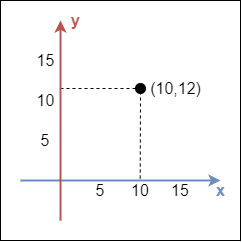### Negative Values

Starting off from the origin, it's possible to head in the opposite direction along each axis (x left, y down) yielding negative values. Thus, we can also have points such as (-10, 12) or even (-10, -12).

It's common to split the Cartesian space into four pieces, which are known as Quadrants. They are denoted by latin numbers in counter-clockwise direction, as shown below.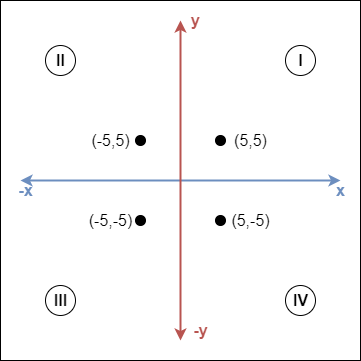• Quadrant I : both x and y are positive
• Quadrant II : x negative, y positive
• Quadrant III : both x and y are negative
• Quadrant IV : x positive, y negative

### More Dimensions

• For one dimension, we use the number line, where the position of a point is a single number.
• For two dimensions we use the 2D Cartesian coordinates, where two numbers are needed. Thus, specifying points as an ordered pair of (x, y) format.
• For three dimensions we use Cartesian coordinates again, but with three axes, the x-, y- and z-axis, where z specifies the depth. Think of it as a somewhat forward-backward motion. The origin is now at (0, 0, 0), and a point is specified as (x, y, z).

Mathematically, we could increase the dimensions even more using that principle, but it's difficult to perceive shapes which can't be seen by us three-dimensional beings.

## Basic Trigonometry

Trigonometry is a branch of mathematics which is all about triangles. It helps us find angles and distances, and has lots of applications.

### Right-Angled Triangles

One of the most interesting triangles is the one with a right angle (angle of 90°). The right angle is shown by a little box in the corner. Additionally, for any other angle θ of the triangle, the sides get specific names:

• Adjacent : next to angle θ
• Opposite : opposite to angle θ
• Hypotenuse : the longest side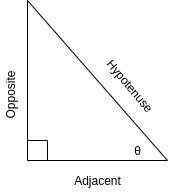### Trigonometric Functions

On a right-angled triangle and for any angle θ (but not the right angle), we can define the three main trigonometric fuctions from the side names, as follows: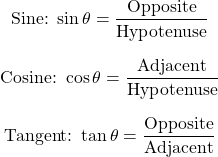For the angles of 30°, 45° and 60°, the values can be easily remembered: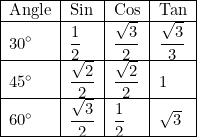• sin goes √1, √2, √3, divided by 2
• cos goes √3, √2, √1 divided by 2
• tangent is the division of sin and cos

In the Four Quadrants, the values of the trigonometric functions have the following sign:

• Quadrant I - all trigonometric functions are positive
• Quadrant II - only sinus positive
• Quadrant III - only tangent positive
• Quadrant IV - only cosinus positive

So, there is a pattern: "A-S-T-C", that is easy to remember.

Trigonometric functions are periodic functions, and thus two different angles can return the same sin, cos or tan value. The following simple relations apply: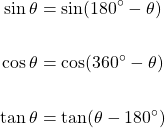We also define inverse trigonometric functions, which based on the sin, cos or tan value, return the angle. These are: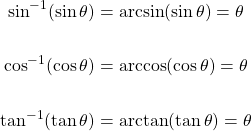### Pythagoras' Theorem

Maybe the most important theorem of Trigonometry is Pythagoras' Theorem, which states:

``````For a right-angled triangle, the square of the long side (hypotenuse) equals the sum of the squares of the other two sides.
``````

or mathematically, if c is the hypotenuse: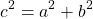### Solving Right Triangles

There are two types of unknown values:

• Unknown angles
• Unknown sides

If the lengths of at least two sides are known, then finding unknown angles is a simple task. If all sides are known, then any trigonometric function can be used. Otherwise, we have to identify the correct trigonometric function to use:

• Opposite and Hypotenuse known → Sinus
• Adjacent and Hypotenuse known → Cosinus
• Adjacent and Opposite known → Tangent

In order to find unknown sides, at least one side and one angle (not including the right angle) has to be known. If two sides are known, then Pythagoras' theorem can be applied directly. If not, then we of course have to choose the correct trigonometric function, so that the known and uknown sides, as well as the known angle are related.

For more information and examples check out part 2 of my trigonometry series.

### Solving Non-Right Triangles

Solving any general triangle is a little more complicated, but it can easily be separated into the following types of problems:

• Three Angles (AAA) → Only calculate the ratio of the sides (can't find the actual size of the triangle)
• Two Angles and a Side not between them (AAS)
• Two Angles and a Side between them (ASA)
• Two Sides and an Angle between them (SAS)
• Two Sides and an Angle not between them (SSA)
• Three Sides (SSS)

For more information on the solving part check out part 3 of my trigonometry series. There I also cover the Law of Sines (or Sine Rule), as well as the Law of Cosines (or Cosine Rule), which play an important role in solving them.

### Trigonometric Equations and Identities

Some Trigonometric Equations and Identities are covered in part 4 of my series. These are basically various useful formulas which can be used in order to relate the trigonometric functions in a more complicated fashion. They are mostly used in Mathematical Analysis.

## RESOURCES:

### Images

Block diagrams and other visualizations were made using draw.io.

## Final words | Next up

And this is actually it for today's post!

In the next part we will get into Circle Theorems and maybe even start with Solid Geometry...

Also, currently, other ideas for "All About" articles that I have in mind include:

• Polynomial Arithmetic
• Exponentials and Logarithms
• Rational Expressions

Basically more High-School Math Refreshers!

See ya!Keep on drifting!

Sort:

I love doing geometry back then but now I work from PC all day but I enjoyed your post